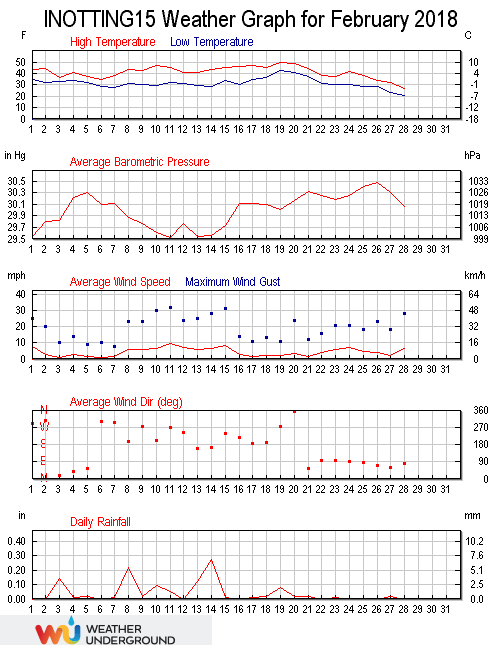February 2018 was much colder than average, with only February 2010 being colder. Rainfall was lower than average, but snowfall was higher. Winds were lighter than average.

The maximum temperature in the month was 10.0°C at 13:48 on the 19th. Only 2010, with a maximum of 8.4°C has seen a lower monthly maximum. The February average maximum is 11.9°C. The minimum temperature in the month of -6.3°C at 02:09 on the 28th, was the lowest since -7.2°C was recorded on February 11th 2012, the second lowest February monthly minimum recorded here. The February average minimum is -3.3°C. The average temperature in the month was 2.3°C, well below the mean of 4.0°C.

The coldest day was the 28th with an average temperature of -5.0°C. The warmest day was the 19th with an average temperature of 8.2°C. On the 28th, the maximum temperature of -2.9°C was the lowest daily maximum temperature ever recorded here, beating the previous lowest of -2.8°C set on December 18th 2010. There were 17 frost days in the month, a record for February.

The highest dew point was 9.1°C at 14:48 on the 19th, with the monthly average dew point being 0.1°C, a February low and 2.0°C below the mean. The lowest dew point was -8.8°C at 18:19 on the 28th, equalling the previous low set in 2012. The high wind chill was 9.8°C set at 17:32 on the 19th, with the low wind chill being -12.4°C set at 22:38 on the 28th. The average wind chill for the month was 0.6°C.

Rainfall for the month totalled 28.4 millimetres, with the wettest day being the 14th when 6.8 millimetres was recorded. This was 7.4 millimetres below the February mean. Rainfall was recorded on 18 days. Snowfall in the month totalled 59 millimetres, 50 millimetres of which fell on the 28th. Measurable snow was noted on 6 days. The highest rain rate was 7.8 millimetres per hour at 15:18 on the 14th, a February low. The rainfall total is 110% of what is expected by the end of February. 87.8 millimetres of rain have now fallen in 2018.

The highest barometric pressure was 1033.6 millibars at 11:22 on the 26th. The lowest barometric pressure was 989.7 millibars at 23:52 on the 10th. The average pressure in the month was 1016.1 millibars. The highest humidity was 100% and the month average humidity was 86%. The lowest humidity was 52% at 15:11 on the 15th.

The highest wind gust in the month was 32 mph recorded at 01:01 on the 11th. The average gust speed in the month was 6.7 mph, mean 7.9 mph. The average wind speed was 4.4 mph, mean 5.1 mph. The maximum wind speed was 19 mph at 01:06 on the 11th, 5.3 mph below the mean. The total wind run was 2878.9 miles, well above the February average of 3447.6 miles, with the predominate direction being 172°.

The maximum high solar radiation recorded at 12:10 on the 27th was 761 w/m², the highest since 766 w/m² was recorded on October 2nd 2017. The average solar radiation for the month was 57 w/m². The highest U.V. Index was 1.2 set at 13:12 on the 25th, the highest since 1.4 was recorded on October 17th 2017.This site uses Akismet to reduce spam. Learn how your comment data is processed.